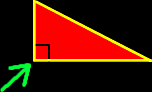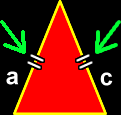# Notations for Triangles

Geometry notations for triangles including the right triangle, congruent sides of a triangle and congruent angles of a triangle

 Notation for a right triangle:The notation for the right angle in a right triangle (or any other right angle) is a little square in the corner.Notation for congruent sides of a triangle:Little tick marks are used to show that two sides are the same length (congruent).  You can use just one tick mark, two or three or more -- just as long as you use the same number on sides that are equal.Notation for congruent angles in a triangle:This works a lot like congruent sides (above)...  You make the little rounded sweep in the angle and then use tick marks to show which angles are the same size (have the same measure -- are congruent).